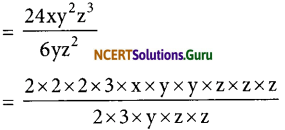# NCERT Solutions for Class 8 Maths Chapter 14 Factorization InText Questions

These NCERT Solutions for Class 8 Maths Chapter 14 Factorization InText Questions and Answers are prepared by our highly skilled subject experts.

## NCERT Solutions for Class 8 Maths Chapter 14 Factorization InText Questions

NCERT Intext Question Page No. 219

Question 1.
Factorise:
(i) 12x + 36
(ii) 22y – 33z
(iii) 14pq + 35pqr
(i) 12x + 36
= (2 x 2 x 3)x + 2 x 2 x 3 x 3
= (2 x 2 x 3) [x + 3]
= 12 (x + 3)(ii) 22y – 33z
= 2 x 11xy – 3 x 11 x z
= 11 (2y – 3z)

(iii) 14pq + 35pqr
= 2 x 7 x p x q + 5 x 7 x p x q x r
= 7pq (2 + 5r)

NCERT Intext Question Page No. 225

Question 1.
Divide:
(i) 24xy2z3 by 6yz2
(ii) 63a2 b4 c6 by 7a2 b2 c3